# How to Count Vowels and Consonants in a String in C

## How to Count Vowels and Consonants in a String in C Language

In this article, I will discuss How to Count Vowels and Consonants in a String in C Language with Examples. Please read our previous article discussing How to Change the Case of Alphabets in a String C Language with Examples.

##### How to Count Vowels and Consonants in a String in C Language?

Now, we will see how to count the number of vowels, consonants, or words in a string. We want to know how many words are there. And also, we want to count the number of vowels and consonants in a string. First, let us understand the vowels and consonants.

To count vowels and consonants in a string in C, you can iterate through each character of the string, check if it’s a vowel or a consonant, and increment the respective counters. Vowels are the characters ‘a’, ‘e’, ‘i’, ‘o’, ‘u’ (and their uppercase counterparts), and consonants are other alphabetic characters. Let us assume we have the following string: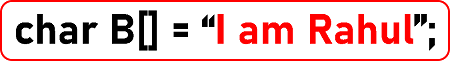So, this is a string “I am Rahul”. As we can clearly see, there are 4 (a, I, u) vowels and 4 (m, R, h, l) consonants. Here, we have taken a string in the form of an array. The above string will be represented in the memory as follows: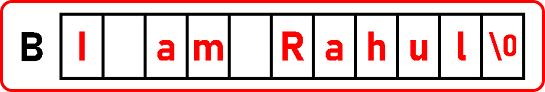Let us see how we can count the vowels in the above string. Here, we will take a vowel counter, let us say, vCount, and if any vowel is found, we increment it. We have to scan the above string and check whether it is a, e, i, o, or u.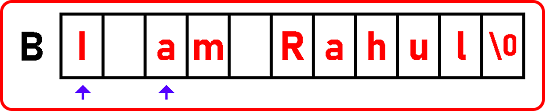Also, we need to check whether the character is uppercase and lowercase. Here, we will write separate conditions. Different conditions for lower cases and upper cases will be written.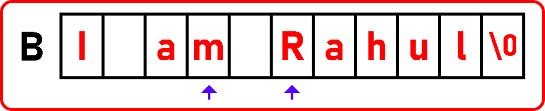If we want to count consonants, we can include one more counter as cCount.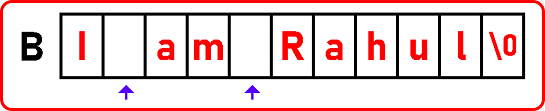Spaces will also be counted, so no special characters or spaces should be taken. We have to pick only the alphabet. So, make sure that it is within the range of the alphabet.

##### Counting Vowels and Consonants in a String Code in C language:
```#include <stdio.h>
#include <stdlib.h>
int main ()
{
char B[] = "I am Rahul";
int i, vCount = 0, cCount = 0;
for (i = 0; B[i] != '\0'; i++)
{
if (B[i] == 'a' || B[i] == 'e' || B[i] == 'i' || B[i] == 'o' || B[i] == 'u' || B[i] == 'A' || B[i] == 'E' || B[i] == 'I' || B[i] == 'O' || B[i] == 'U')
{
vCount++;
}
else if ((B[i] >= 65 && B[i] <= 90) || (B[i] >= 97 && B[i] <= 122))
{
cCount++;
}
}
printf ("String is \"%s\"\n", B);
printf ("Vowels: %d\n", vCount);
printf ("Consonants: %d\n", cCount);
}```
###### Output: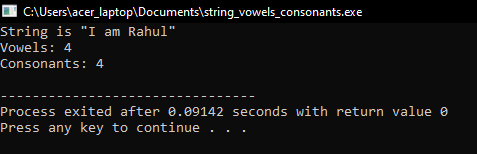Here’s another program to find the Vowels and Consonants in a String. Here, we use the tolower method to convert a character into lowercase for easy comparison, which belongs to #include <ctype.h> header file.

```#include <stdio.h>
#include <ctype.h> // for tolower function

int isVowel(char ch) {
ch = tolower(ch); // Convert to lowercase for easy comparison
return (ch == 'a' || ch == 'e' || ch == 'i' || ch == 'o' || ch == 'u');
}

int main() {
char str[] = "I am Rahul";
int vCount = 0, cCount = 0;

for(int i = 0; str[i] != '\0'; i++) {
if(isalpha(str[i])) { // Check if the character is an alphabet
if(isVowel(str[i])) {
vCount++;
} else {
cCount++;
}
}
}

printf ("String is \"%s\"\n", str);
printf ("Vowels: %d\n", vCount);
printf ("Consonants: %d\n", cCount);

return 0;
}
```
###### In this program:
• The isVowel function checks if a character is a vowel. It converts the character to lowercase for easier comparison.
• The main function iterates over each character of the string str. It uses the isalpha function from ctype.h to check if the character is an alphabetic character.
• If the character is alphabetic, it’s further checked if it’s a vowel using the isVowel function. If it’s a vowel, the vCount counter is incremented; otherwise, the cCount counter is incremented.
• After the loop, it prints the count of cCount and cCount.

This program counts only alphabetic vowels and consonants, ignoring digits, punctuation, and other non-alphabetic characters.

##### Counting Words in a String in C Language:

Counting words in a string in C involves iterating through the string and identifying word boundaries. Typically, a word is defined as a sequence of characters separated by spaces, punctuation, or other delimiters. Next, let us see how to count the number of words in a string. Please observe the following string.How many words are there? 3 words and 2 spaces are there. It means spaces will help us to identify words. So, count the spaces and plus 1. Then, we get the number of words in a string. Let us modify the above string as follows: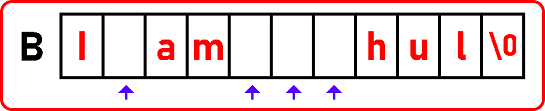Still, there are 3 words, but the number of spaces is 4, and if we add one, it will be 5. And 5 is not the right answer. Here, we have to deal with excess space. So, when you have a convenient set of spaces, it is actually called white space. So, if there are any white spaces, we should check that also.

So, let us see how to do that. Check that a previous one is also a space whenever we find a space. If so, then count that. Below is the code for finding the total number of words in a string:

##### Counting words in a String Code in C language:
```#include <stdio.h>
#include <stdlib.h>
int main ()
{
char B[] = "I am   Rahul";
int i, word = 1;
for (i = 0; B[i] != '\0'; i++)
{
if (B[i] == ' ' && B[i - 1] != ' ')
{
word++;
}
}
printf ("String is \"%s\"\n", B);
printf ("Total Words: %d\n", word);
}```
###### Output: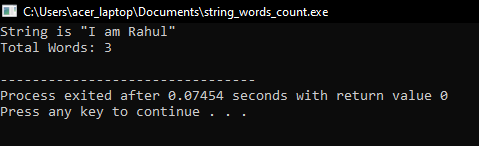##### Another Way to Count the Words in a String:
```#include <stdio.h>
#include <ctype.h> // for isspace function

int countWords(const char *str) {
int inWord = 0;
int wordCount = 0;

while (*str) {
if (isspace((unsigned char)*str)) {
inWord = 0;
} else if (!inWord) {
inWord = 1;
wordCount++;
}
str++;
}

return wordCount;
}

int main() {
char str[] = "I am   Rahul";
int words = countWords(str);

printf ("String is \"%s\"\n", str);
printf ("Total Words: %d\n", words);

return 0;
}```

In this program:

• The countWords function takes a string and iterates through it.
• isspace checks if the current character is a space or other whitespace character (like tabs or newlines).
• inWord is a flag that indicates whether the current position is inside a word. This flag helps in identifying the start of a new word.
• When transitioning from a space to a non-space character, the function increments wordCount, signaling the start of a new word.
• The main function calls countWords with a test string and prints the result.